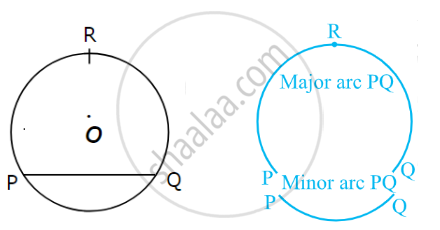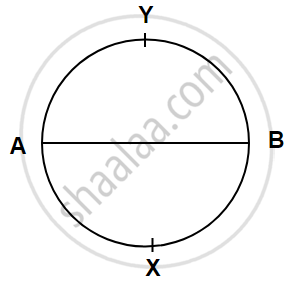# Arc of the Circle

#### definition

Arc of the Circle: A chord divides the circumference of a circle into two parts and each part is called an arc.

Minor Arc: The arc which is less than the semicircle is called minor arc.

Major Arc: The arc which is greater than the semicircle is called major arc.

# Arc of the Circle:• An arc is a part of the circumference of a circle.

• A chord divides the circumference of a circle into two parts and each part is called an arc.

• In the figure, given above, chord PQ divides the circumference into two unequal arc PRQ and arc PQ.

• The arc which is less than the semicircle is called minor arc.

• The arc which is greater than the semicircle is called major arc.

• If two arcs of a circle have common endpoints and the arcs make one complete circle, the arcs are said to be corresponding arcs. Here, arc PRQ and arc PQ are mutually corresponding arcs.

• In the figure below, chord AB is a diameter of the circle. The diameter gives rise to two equal arcs. They are called semicircular arcs.If you would like to contribute notes or other learning material, please submit them using the button below.

### Shaalaa.com

Arc of the Circle [00:02:26]
S
0%